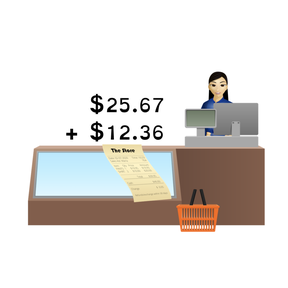Adding decimal numbers to 100 using the Standard Algorithm

# Adding decimal numbers to 100 using the Standard Algorithm

I can add decimals to 100 using the standard algorithm.8,000 schools use Gynzy92,000 teachers use Gynzy1,600,000 students use Gynzy

## General

Students will learn to add decimal numbers to 100 using the standard algorithm. They will understand the importance of lining up decimal points and filling the empty spaces after the decimal with zero before they add. They will apply these skills to solving word problems.

## Standards

CCSS.Math.Content.6.NS.B.3

## Learning objective

Students will be able to add decimals to 100 using the standard algorithm.

## Introduction

Students will review the names of the 3 places after the decimal point. They will practice saying the decimal numbers out loud.

## Instruction

Students are instructed on how to line up decimals with the visual of numbers written on grid paper. Next, they are shown to fill in missing numbers after the decimal with zeros before they add. They are reminded to place the decimal in the final answer after adding. The students will copy several problems on their own to practice lining up and placing decimals in their answers. They are shown that adding money amounts includes working with decimals. Further practice is provided with word problems, including some adding money amounts. Finally, they are shown two addition problems with errors. They will tell how to complete them correctly.

## Quiz

Students respond to ten multiple-choice and true/false questions.

## Closing

Students will determine the decimal numbers represented on a grid and add them up.

## Instruction materials

Students are encouraged to copy and solve problems, so paper and pencil is suggested.

### The online teaching platform for interactive whiteboards and displays in schools

• Save time building lessons

• Manage the classroom more efficiently

• Increase student engagement